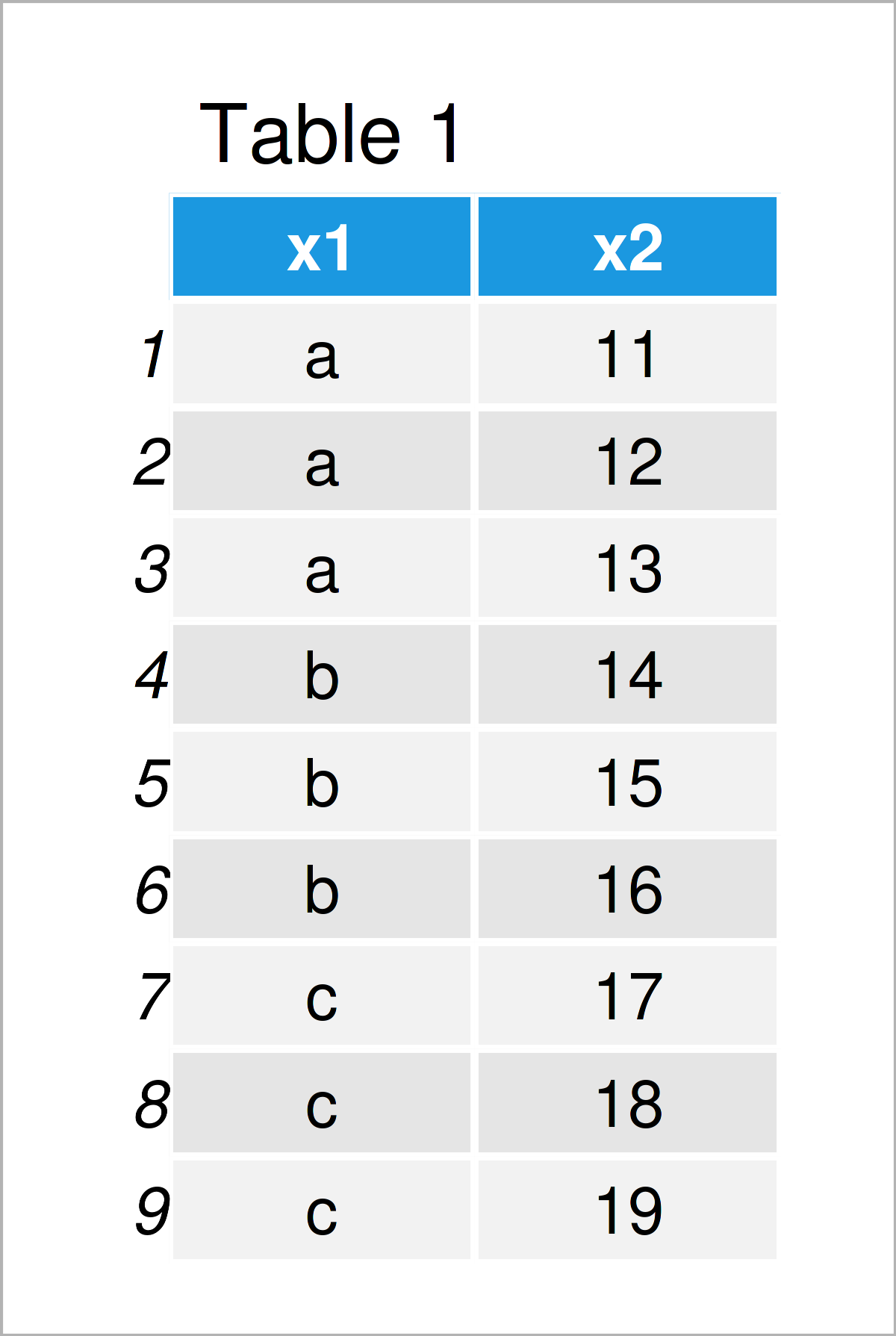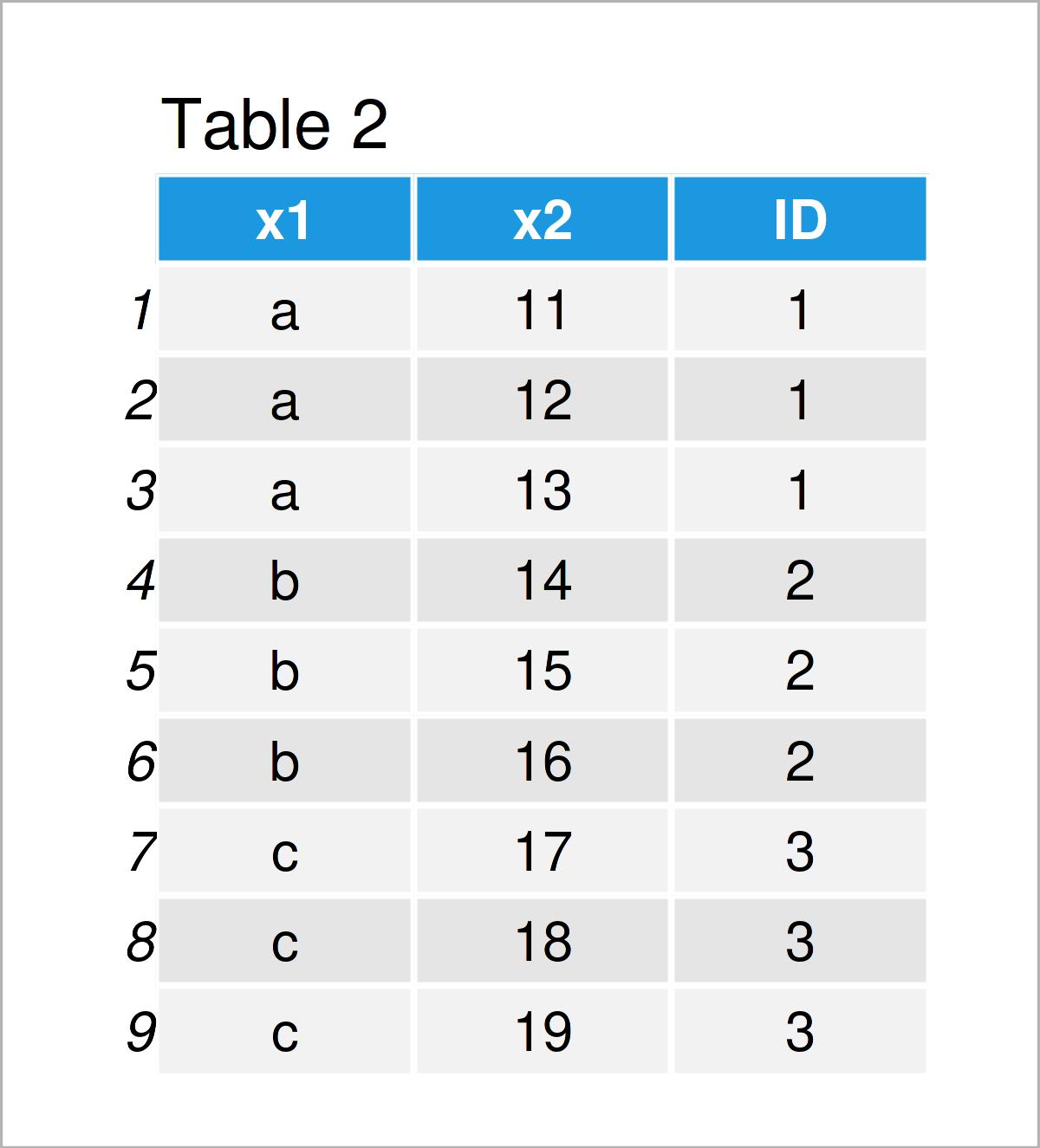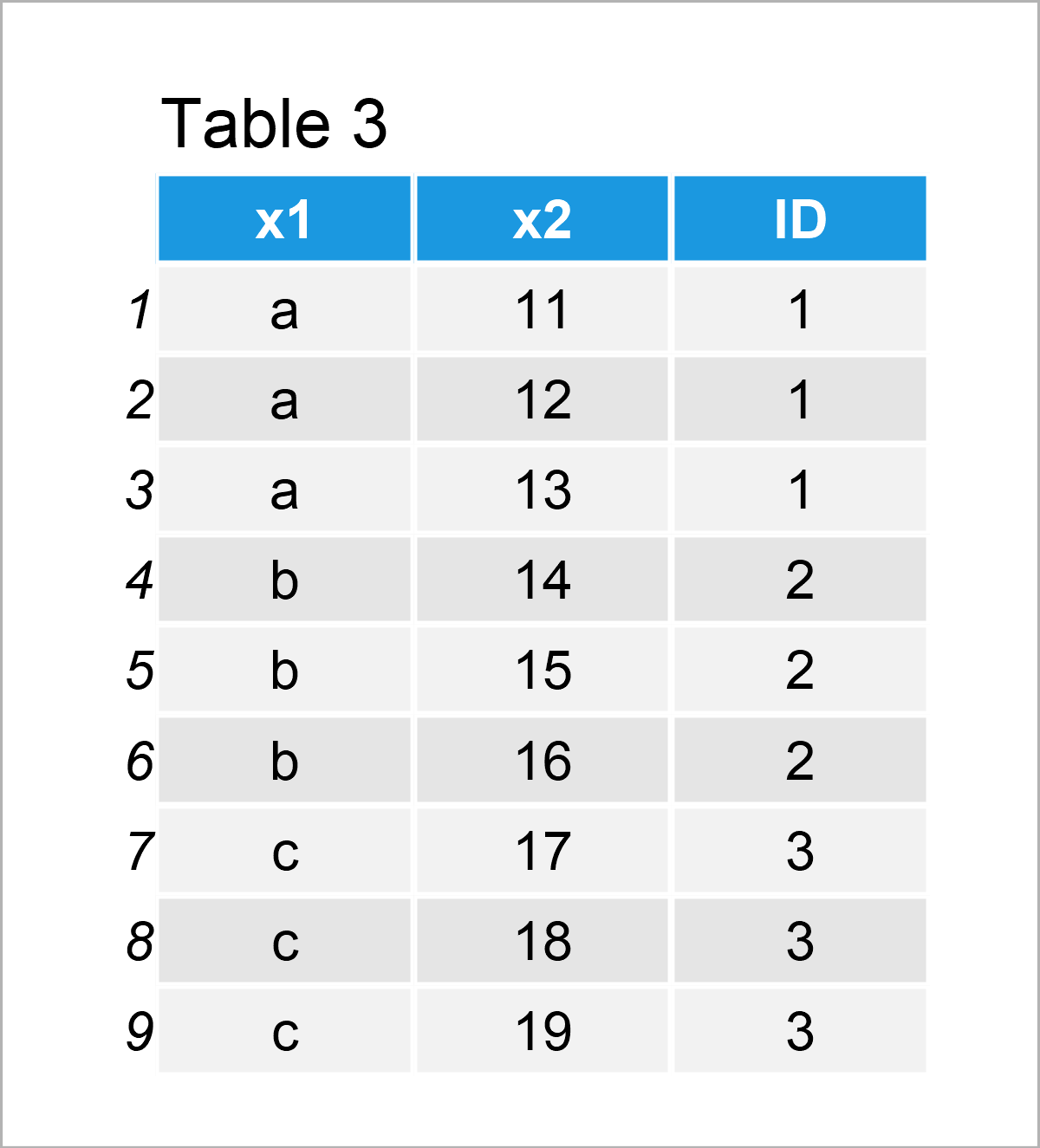# Assign Unique ID for Each Group in R (3 Examples)

In this R tutorial you’ll learn how to create an ID number by group.

The article will consist of this content:

Here’s how to do it…

## Creation of Example Data

We’ll use the following data as basement for this R programming tutorial:

```data <- data.frame(x1 = rep(letters[1:3],                   # Create example data
each = 3),
x2 = 11:19)
data                                                        # Print example data```Have a look at the table that got returned by the previous R syntax. It shows that our example data has nine lines and two variables. The variable x1 has the character data type and the variable x2 has the integer class.

## Example 1: Add Consecutive Group Number to Data Frame Using Base R

In this example, I’ll demonstrate how to group by a variable and then assign an ID based on these groups using the transform function of the basic installation of the R programming language.

Have a look at the following R code:

```data_id1 <- transform(data,                                 # Create ID by group
ID = as.numeric(factor(x1)))
data_id1                                                    # Print data with group ID```After executing the previous R programming code the data frame with consecutive ID column illustrated in Table 2 has been created.

## Example 2: Add Consecutive Group Number to Data Frame Using dplyr Package

In this example, I’ll demonstrate how to create a unique ID column by group using the dplyr package.

First, we need to install and load the dplyr package:

```install.packages("dplyr")                                   # Install & load dplyr package
library("dplyr")```

Next, we can use the group_by and mutate functions of the dplyr package to assign a unique ID number to each group of identical values in a column (i.e. x1):

```data_id2 <- data %>%                                        # Create ID by group
group_by(x1) %>%
dplyr::mutate(ID = cur_group_id())
data_id2                                                    # Print data with group ID
# # A tibble: 9 x 3
# # Groups:   x1 
#   x1       x2    ID
#   <chr> <int> <int>
# 1 a        11     1
# 2 a        12     1
# 3 a        13     1
# 4 b        14     2
# 5 b        15     2
# 6 b        16     2
# 7 c        17     3
# 8 c        18     3
# 9 c        19     3```

By running the previous R programming syntax we have created the tibble shown in the previous RStudio console output.

## Example 3: Add Consecutive Group Number to Data Frame Using data.table Package

In this example, I’ll demonstrate how to use the data.table package to add a unique ID variable by group.

We first have to install and load the data.table package:

```install.packages("data.table")                              # Install data.table package

Next, we can use the functions of the data.table package to assign an ID number to our data:

```data_id3 <- data                                            # Duplicate data
setDT(data_id3)[ , ID := .GRP, by = x1]                     # Create ID by group
data_id3                                                    # Print data with group ID```After executing the previous R code, the data set with ID column shown in Table 3 has been created. Note that this data set has the data.table class.

## Video, Further Resources & Summary

I have recently published a video on my YouTube channel, which illustrates the contents of this tutorial. You can find the video below:

Please accept YouTube cookies to play this video. By accepting you will be accessing content from YouTube, a service provided by an external third party.If you accept this notice, your choice will be saved and the page will refresh.

Furthermore, you could have a look at the related posts on my homepage. Some tutorials about topics such as counting, groups, extracting data, and numeric values are listed below.

Summary: In this R tutorial you have learned how to add a consecutive ID number by group. Don’t hesitate to let me know in the comments, if you have any additional questions.

Subscribe to the Statistics Globe Newsletter